# 吴恩达机器学习4

## 神经网络

### 神经元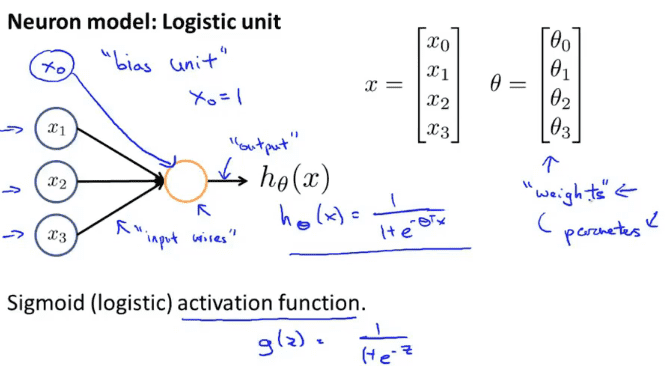$\theta$在之前的描述种被称为模型的参数，在神经网络种有人会称它为模型的权重

### 神经网络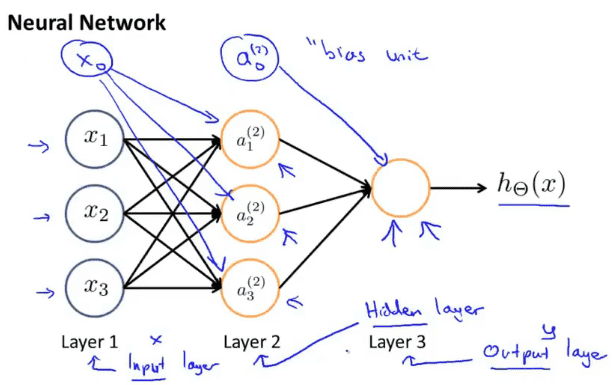#### 计算步骤

$a_i^{(j)}$代表第$j$层第$i$个神经元或单元

$\Theta^{(j)}$ 权重参数，控制从某一层到下一层的映射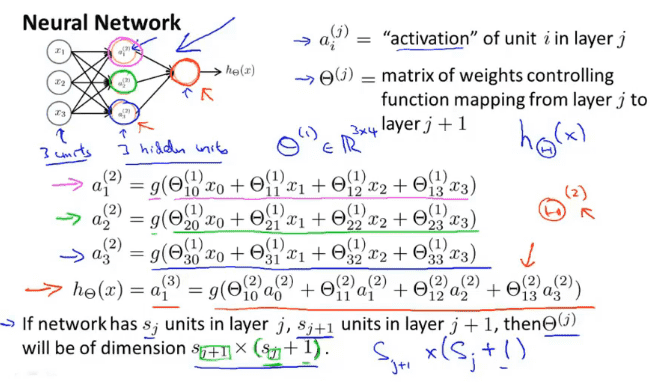### 向量化表示

$z^{(2)}$由$\Theta^{(1)}$与$a^{(1)}$矩阵相乘而来，$a^{(1)}$时输入的参数，加上隐藏的$x_0$的话，$a^{(1)}$的规格时$4\times 1$，以此类推，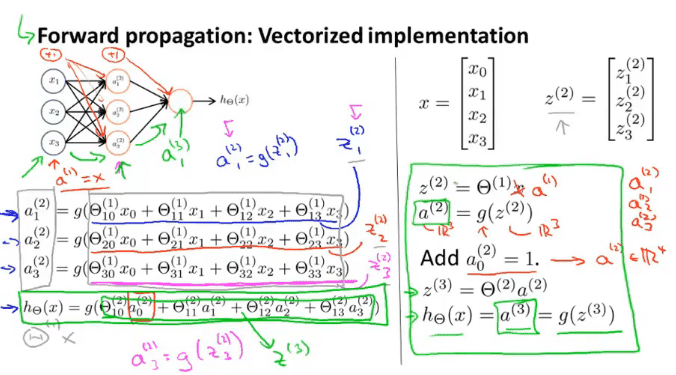### 神经元计算样例

#### 与运算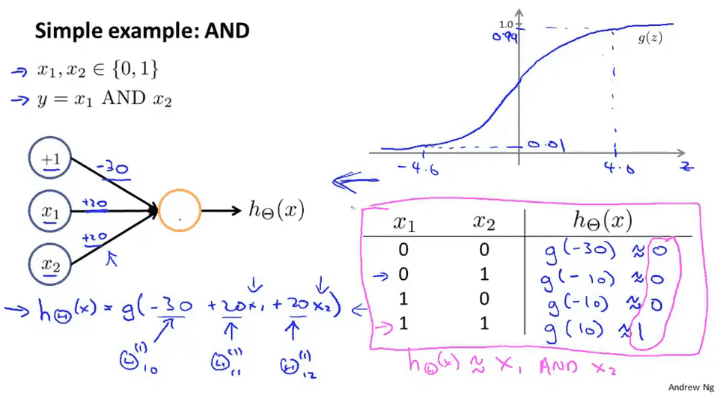#### 或运算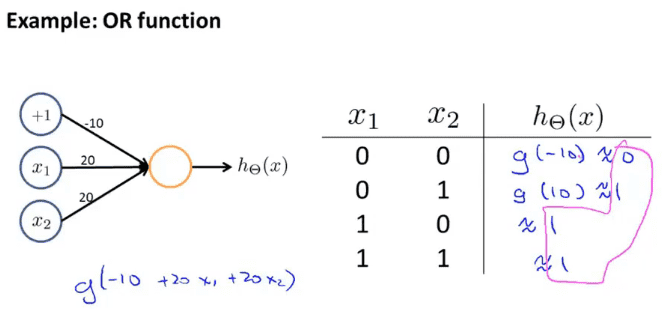#### 非运算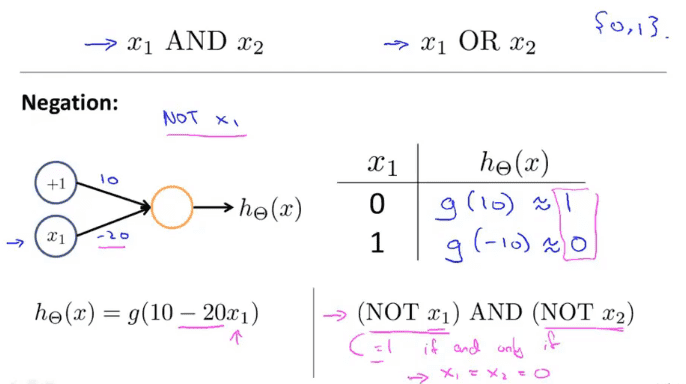#### 异或运算.32ylofm62j40.png)

### 多元分类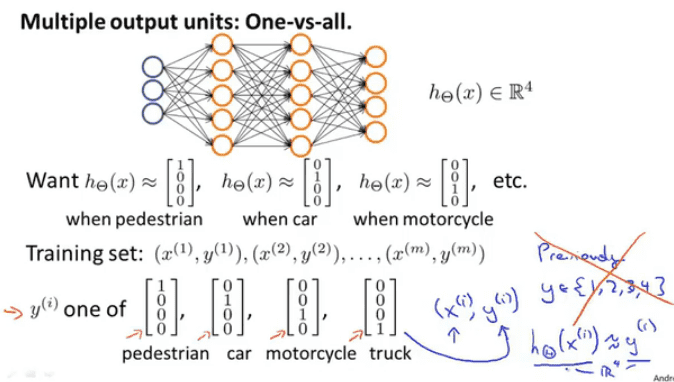目录